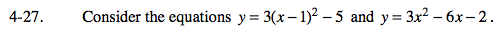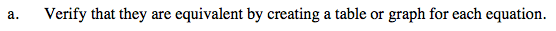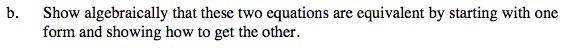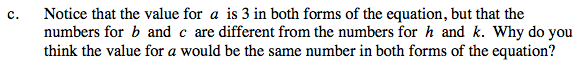### Home > A2C > Chapter Ch4 > Lesson 4.1.2 > Problem4-27

4-27.
1. Consider the equations y = 3(x − 1)2 − 5 and y = 3x2 − 6x − 2. 4-27 HW eTool (Desmos). Homework Help ✎

1. Verify that they are equivalent by creating a table or graph for each equation.

2. Show algebraically that these two equations are equivalent by starting with one form and showing how to get the other.

3. Notice that the value for a is 3 in both forms of the equation, but that the numbers for b and c are different from the numbers for h and k. Why do you think the value for a would be the same number in both forms of the equation?If your tables or graphs are not equivalent, you may have made a mistake. Make sure to check your work.y = 3(x − 1)2 − 5
y = 3(x2 − 2x + 1) − 5
y = 3x2 − 6x + 3 − 5
y = 3x2 − 6x − 2

Note: there are many ways to solve this.Look at the method for changing one equation into the other. Pay close attention to the value of a.

Use the eTool below to graph the equations.
Click the link at right for the full version of the eTool: A2C 4-27 HW eTool